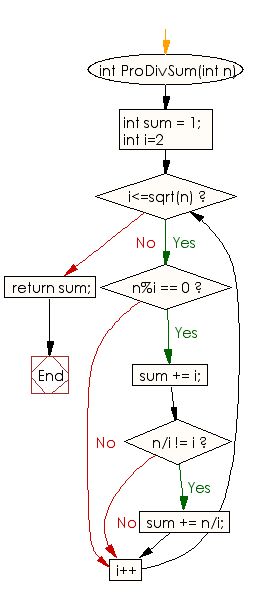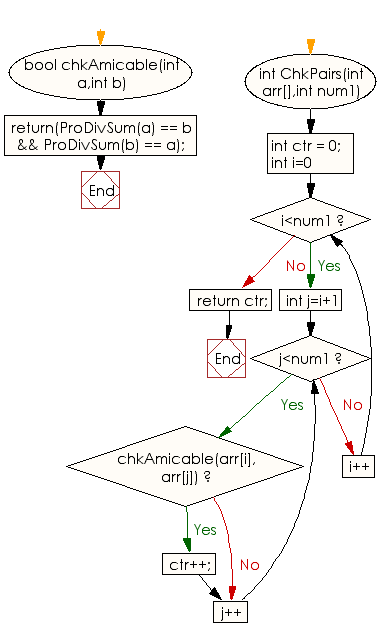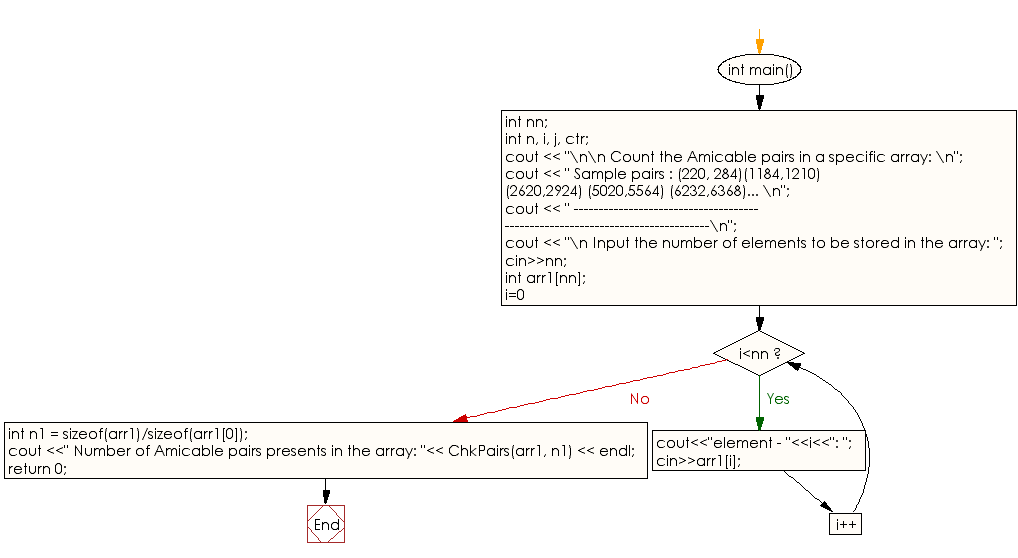﻿ C++ Exercises: Count the Amicable pairs in an array - w3resource

# C++ Exercises: Count the Amicable pairs in an array

## C++ Numbers: Exercise-29 with Solution

Write a C++ program to count the amicable pairs in an array.

Sample Solution:

C++ Code :

``````#include <bits/stdc++.h>
using namespace std;

int ProDivSum(int n)
{
int sum = 1;
for (int i=2; i<=sqrt(n); i++)
{
if (n%i == 0)
{
sum += i;

// To handle perfect squares
if (n/i != i)
sum += n/i;
}
}
return sum;
}
bool chkAmicable(int a,int b)
{
return(ProDivSum(a) == b && ProDivSum(b) == a);
}
int ChkPairs(int arr[],int num1)
{
int ctr = 0;
for (int i=0; i<num1; i++)
for (int j=i+1; j<num1; j++)
if (chkAmicable(arr[i], arr[j]))
ctr++;
return ctr;
}
int main()
{
int nn;
int n, i, j, ctr;
cout << "\n\n Count the Amicable pairs in a specific array: \n";
cout << " Sample pairs : (220, 284)(1184,1210) (2620,2924) (5020,5564) (6232,6368)... \n";
cout << " ------------------------------------------------------------------------------\n";
cout << "\n Input the number of elements to be stored in the array: ";
cin>>nn;
int arr1[nn];
for(i=0;i<nn;i++)
{
cout<<"element - "<<i<<": ";
cin>>arr1[i];
}
int n1 = sizeof(arr1)/sizeof(arr1);
cout <<" Number of Amicable pairs presents in the array: "<< ChkPairs(arr1, n1) << endl;
return 0;
}
``````

Sample Output:

```Count the Amicable pairs in a specific array:
Sample pairs : (220, 284)(1184,1210) (2620,2924) (5020,5564) (6232,6368)...
------------------------------------------------------------------------------

Input the number of elements to be stored in the array: 2
element - 0: 220
element - 1: 284
Number of Amicable pairs presents in the array: 1
```

Flowchart:C++ Code Editor:

What is the difficulty level of this exercise?

﻿

## C++ Programming: Tips of the Day

How to Use CCache with CMake?

This directory contains loads of symlinks for every possible name the compiler could be called from (like gcc and gcc-4.3), all pointing to ccache.

And I didn't even create the symlinks. That directory comes pre-filled when I install ccache on Debian.

Ref: https://bit.ly/3KoJN3g

We are closing our Disqus commenting system for some maintenanace issues. You may write to us at reach[at]yahoo[dot]com or visit us at Facebook